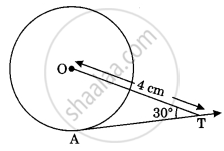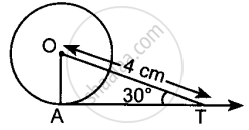# In figure, AT is a tangent to the circle with centre O such that OT = 4 cm and ∠OTA = 30°. Then AT is equal to ______ - Mathematics

MCQ
Fill in the Blanks

In figure, AT is a tangent to the circle with centre O such that OT = 4 cm and ∠OTA = 30°. Then AT is equal to ______#### Options

• 4 cm

• 2 cm

• 2sqrt3 cm

• 4sqrt3 cm

#### Solution

In figure, AT is a tangent to the circle with centre O such that OT = 4 cm and ∠OTA = 30°. Then AT is equal to underline(2sqrt3) cm.

Explanation:

∠OAT = 30°  ..........[∵ Tangent and radius are ⊥ to each other at the point of contact]In right-angled ΔOAT,

"AT"/"OT" = cos 30°

⇒ "AT"/4 = sqrt3/2 ⇒ AT = 2sqrt3 cm.

Concept: Tangent to a Circle
Is there an error in this question or solution?
Chapter 9: Circles - Exercise 9.1 [Page 103]

#### APPEARS IN

NCERT Mathematics Exemplar Class 10
Chapter 9 Circles
Exercise 9.1 | Q 6 | Page 103

Share